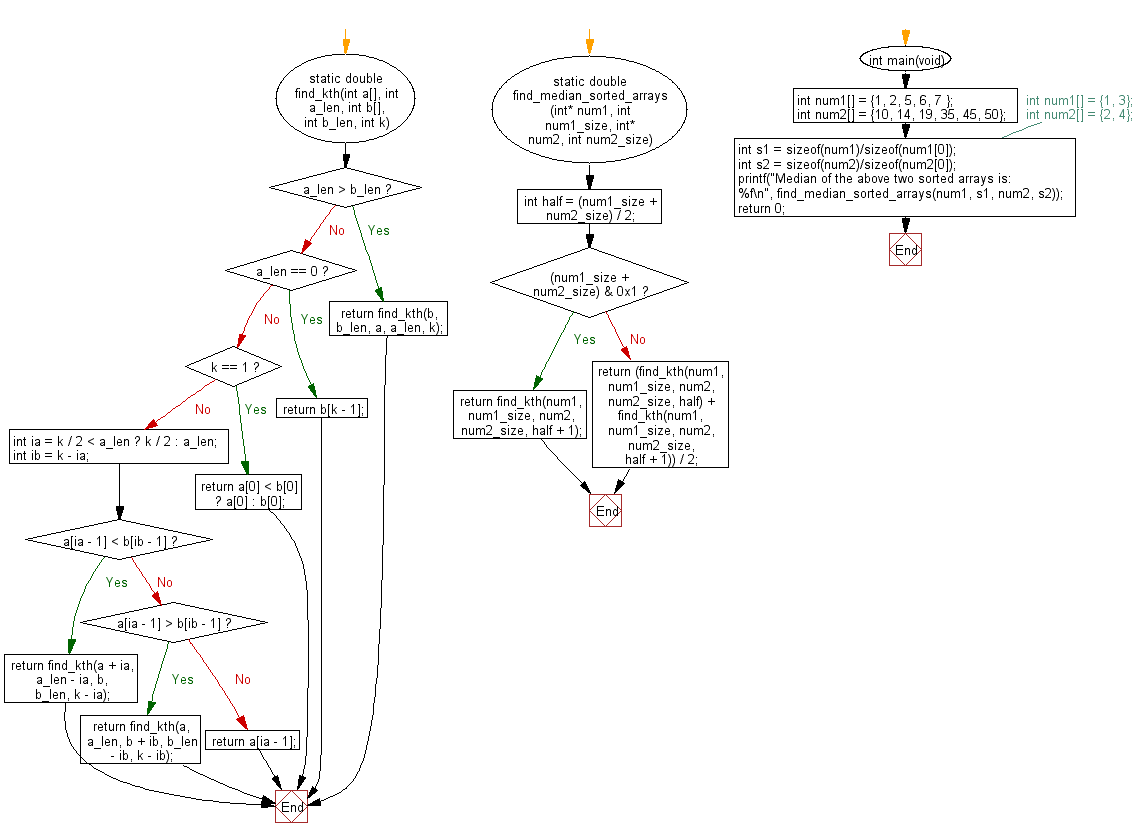﻿ C Program: Median of two non-empty sorted arrays# C Exercises: Find the median of the two given sorted arrays which are not empty

## C Programming Practice: Exercise-3 with Solution

Write a C programming to find the median of the two given sorted arrays which are not empty.

C Code:

``````#include <stdio.h>
#include <stdlib.h>
static double find_kth(int a[], int a_len, int b[], int b_len, int k)
{
if (a_len > b_len) {
return find_kth(b, b_len, a, a_len, k);
}

if (a_len == 0) {
return b[k - 1];
}

if (k == 1) {
return a < b ? a : b;
}

int ia = k / 2 < a_len ? k / 2 : a_len;
int ib = k - ia;
if (a[ia - 1] < b[ib - 1]) {

return find_kth(a + ia, a_len - ia, b, b_len, k - ia);
}
else if (a[ia - 1] > b[ib - 1]) {
return find_kth(a, a_len, b + ib, b_len - ib, k - ib);
} else {
return a[ia - 1];
}
}

static double find_median_sorted_arrays(int* num1, int num1_size, int* num2, int num2_size)
{
int half = (num1_size + num2_size) / 2;
if ((num1_size + num2_size) & 0x1) {
return find_kth(num1, num1_size, num2, num2_size, half + 1);
} else {
return (find_kth(num1, num1_size, num2, num2_size, half) + find_kth(num1, num1_size, num2, num2_size, half + 1)) / 2;
}
}
int main(void)
{
int num1[] = {1, 2, 5, 6, 7 };
int num2[] = {10, 14, 19, 35, 45, 50};
//int num1[] = {1, 3};
//int num2[] = {2, 4};
int s1 = sizeof(num1)/sizeof(num1);
int s2 = sizeof(num2)/sizeof(num2);
printf("Median of the above two sorted arrays is: %f\n", find_median_sorted_arrays(num1, s1, num2, s2));
return 0;
}
``````

Sample Output:

```Median of the above two sorted arrays is: 10.000000
```

Flowchart:## C Programming Code Editor:

What is the difficulty level of this exercise?

Test your Programming skills with w3resource's quiz.

﻿

## C Programming: Tips of the Day

Maximum value of int:

In C:

```#include <limits.h>
then use
int imin = INT_MIN; // minimum value
int imax = INT_MAX;```

or

```#include <float.h>

float fmin = FLT_MIN;  // minimum positive value
double dmin = DBL_MIN; // minimum positive value

float fmax = FLT_MAX;
double dmax = DBL_MAX;```

Ref : https://bit.ly/3fi8yk9